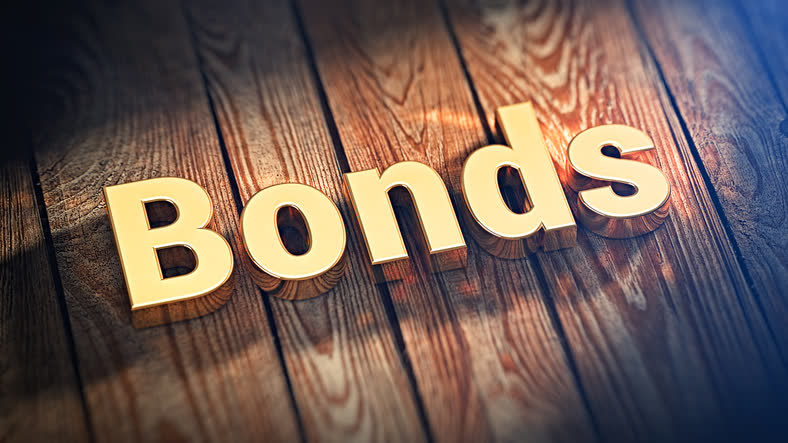Select Page

# Explain Bonds, Bond Terms, Price and Yield, Types of Bond Risk## Explain Bonds

A bond is a security representing a loan. It is a liability for the issuer (usually a government or company), and an asset for the bondholder (usually an entity or individual investor). A bond holder is an individual or entity that has loaned money to a bond issuer.

## Bond Terms

### Par Value

Par value is the amount of money (usually \$1000 per bond) that will be returned to the bondholder at the maturity date.

### Coupon

The interest the bond pays annually.

### Coupon Rate

The coupon rate is the coupon (interest) divided by par value. If a bond pays \$30 annually it has a coupon rate of 3.0% (\$30 divided by \$1,000).

### Maturity

Maturity is the length of time before the bond issuer pays the par value to the bond holder. Obviously, the maturity decreases as time passes.

### Duration

Duration is a complicated calculation but an important risk measurement. Duration is the weighted average time period of the present value of cash flows (interest and principle payments).

Zero coupon bonds will have a duration equal to the maturity because all cash flows are received at maturity. Bonds that pay interest will have a duration less than maturity because of interest payments paid before maturity. The higher the coupon the shorter the duration because more cash flows are received early.

### Bond Price and Yield

If the bondholder purchased a bond at par when issued, the price would be \$1,000 and the yield would be the coupon rate. But most investors purchase bonds at a price over or below par value. Many of these purchases are made on the secondary market where bonds are traded regularly (similar to a stock exchange), making buying and selling bonds easy.

The market price of a bond will most likely be more or less than the par value (the amount paid the bondholder at maturity). The bondholder will still receive the coupon or interest (usually semi-annually) and the par value at maturity.

### Current Yield

Current yield is the coupon (interest) divided by the current market price of the bond, which maybe more or less than the par value.

### Yield to Maturity

Yield to Maturity is the amount of return (expressed as a percentage) a bondholder will make on the bond if purchased at market value and held to maturity. This is a complicated calculation usually supplied by the bond broker or exchange.

The yield to maturity takes into account the coupon paid until maturity and the difference between the market price of the bond and par value. If the market price is lower than par value, ,the yield to maturity will be higher than the coupon rate because the bondholder will receive more money (par value) at maturity than the price paid for the bond. If the market price is higher than par value, the yield to maturity will be lower than the coupon rate because the bondholder will receive less money (par value) than the price paid for the bond.

## Types of Bond Risk

Bonds have two main types of bond risk that every investor should understand before purchasing a bond (making a loan).

### Credit Risk

Credit risk is the risk that the issuer will be unable to pay the coupon (interest) and/or par value at maturity. The financial position of an issuing entity can change over time, affecting the price of the bond before maturity.

### Interest Rate Risk

The price of a bond moves in the opposite direction of bond yields. Since the coupon (interest) on the bond is fixed, the price of the bond will rise or fall to provide a yield to maturity on the bond equal to the current market rate required by investors.

The price change of a bond will approximate the change in interest rate times the duration of the bond. For example, if interest rates rise 2% and an investor owns a bond with an 8 year duration, the price of the bond will decline by approximately 16%. A bond with a long duration will be much more volatile than a bond with a short duration when interest rates change.

## Bond Basics

Now you understand the basics: what a bond is, bond terms, the relationship between price and yield, and the two main risks of owning bonds.

Don’t let the past several decades be your guide regarding bond risks and returns. The period starting in 1980 saw yields fall from record highs to record lows. That means current prices are near record highs and offer near record low yields.

There is no rule that you must have bonds in your portfolio. Remember, as bond yields rise, bond prices fall. Always consider the current risk of a bond or bond fund before including it in your portfolio.